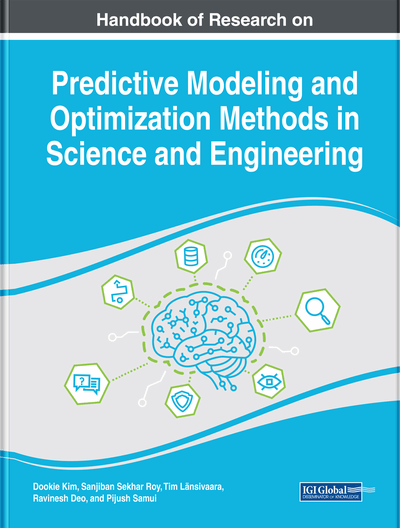# Optimization of the Angle of Twist of Propeller Using Modified Flower Pollination Algorithm

Kok Meng Ong (Universiti Tun Hussein Onn Malaysia, Malaysia), Pauline Ong (Universiti Tun Hussein Onn Malaysia, Malaysia) and Chee Kiong Sia (Universiti Tun Hussein Onn Malaysia, Malaysia)
DOI: 10.4018/978-1-5225-4766-2.ch014
Available
\$37.50
No Current Special Offers

## Abstract

In this chapter, a real-world mechanical engineering design problem (i.e., determining the angle of twist of propeller in order to maximize its thrust) was solved using modified flower pollination algorithm (MFPA). First, the relationship between angle of twist and the propeller thrust is explained. Then, the amount of experiments needed for this problem is determined using Box-Behnken design, whereas the mathematical model of this problem is developed using response surface methodology. After that, the overview of flower pollination algorithm (FPA) and its limitation is explained in detail. A proposed MFPA based on chaos theory, frog leaping local search, and inertia weight is explained next. The procedure of solving this problem is explained and the result of this problem is shown later with validation. The optimal solutions obtained from MFPA are 89°, 50.9730°, 48.4565°, 44.6729°, and 64.2286° for five angles of twist start from hub until the tip of the blade.
Chapter Preview
Top

## Introduction

Bernoulli’s principle states that the speed of a moving fluid is inversely proportional to pressure within the fluid. When the airfoil is subjected to a relative wind as illustrated in Figure 1, the speed of a moving fluid is higher at the top of the airfoil, as compared to the bottom of the airfoil. Thus, the pressure within the fluid is higher at the bottom according to Bernoulli’s principle. Eventually, higher pressure at the bottom of the airfoil causes a lift force pushes the airfoil upward.

Angle of attack, α, is the angle between the chord line of an airfoil and the relative wind, as shown in Figure 2. From the experimental result, researcher found that the lift coefficient of a fixed-airfoil varies with angle of attack. The graph in Figure 3 shows that the increasing of angle of attack of the airfoil will increase the lift coefficient up to the maximum lift coefficient. After that, the lift coefficient decreases due to a noticeable separation of the airflow from the upper surface of the airfoil.

As illustrated in Figure 4, a propeller is an example of open axial-flow fans. In reality, there are some twists in the propeller blade due to different pitch angles at any radius of the propeller. Pitch angle is the angle between the chord line of an airfoil and the horizontal axis. As shown in this figure, the pitch angle at the cross section near the hub () is higher than the pitch angle at the cross section near the tip (). This is due to the tangential speed of the blade increases linearly with the radius which follow the equation as below.

(1)(2) where the value ofis equal to the tangential blade speed,. As shown in Figure 5, the tangential blade speed is pointed towards the left. The relative velocity,of the air can be graphically drawn as shown in Figure 5. Angle of attack, is the same along the propeller blade in order to obtain maximum coefficient of lift. Therefore, there are some twists along the blade such that is the same at any radius.

## Key Terms in this Chapter

Angle of Attack: It is the angle between the chord line of an airfoil and the relative wind.

Cross-Pollination: It is a pollination process that including different species of flowers.

Biotic Form of Pollination: There are pollinators to transfer the pollen in flower pollination such as beetle, fly, and bee.

Self-Pollination: It is a pollination process that including same species of flowers.

Abiotic Form of Pollination: There are no pollinators to transfer the pollen in flower pollination. It depends on natural phenomena such as wind and water to transfer the pollen from one flower to another.

Angle of Twist: It is the angle along the blade such that angle of attack is the same at any radius.

Optimization: It is a process of finding the best combination of design variables that achieve the objective of the problem without evaluation on all possible combination.

Flower Pollination Algorithm: It is a metaheuristic optimization algorithm developed by Xin-She Yang based on aforesaid characteristics of flower pollination.

Bernoulli’s Principle: This principle states that an increase in the speed of a moving fluid simultaneously with a decrease in pressure within the fluid.

## Complete Chapter List

Search this Book:
Reset